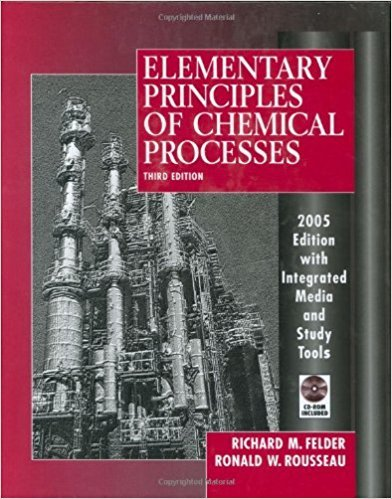×
Get Full Access to Elementary Principles Of Chemical Processes - 3 Edition - Chapter 9 - Problem 9.11
Get Full Access to Elementary Principles Of Chemical Processes - 3 Edition - Chapter 9 - Problem 9.11

×

# n-Butane is converted to isobutane in a continuousISBN: 9780471687573 143

## Solution for problem 9.11 Chapter 9

Elementary Principles of Chemical Processes | 3rd Edition

• Textbook Solutions
• 2901 Step-by-step solutions solved by professors and subject experts
• Get 24/7 help from StudySoup virtual teaching assistantsElementary Principles of Chemical Processes | 3rd Edition

4 5 1 324 Reviews
19
3
Problem 9.11

n-Butane is converted to isobutane in a continuous isomerization reactor that operates isothermally at 149C. The feed to the reactor contains 93 mole% n-butane, 5% isobutane, and 2% HCI at 149C, and a 40% conversion of n-butane is achieved. (a) Taking a basis of 1 mol of feed gas, calculate the moles of each component of the feed and product mixtures and the extent of reaction, ~(mol). (b) Calculate the standard heat of the isomerization reaction (kllmol). Then, taking the feed and product species at 25C as references, prepare an inlet-outlet enthalpy table and calculate and fill in the component amounts (mol) and specific enthalpies (kllmol). (See Example 9.5-1.) (c) Calculate the required rate of heat transfer (kl) to or from the reactor (state which it is). Then determine the required heat transfer rate (kW) for a reactor feed of 325 moUh. (d) Use your calculated results to determine the heat of the isomerization reaction at 149C, IlHr(149C)(kllmol).

Step-by-Step Solution:
Step 1 of 3

Chemistry Chapter 1 What is Chemistry  Chemistry­ the overall study of matter and the transformations in can undergo.  Matter­ anything that has mass and takes up space. o Sound, Light, and Heat take up 0 space and have no mass therefore deeming them to have NO MATTER. Research  Basic Research­ research used to further knowledge about how the natural world operates.  Applied Research­ the use of prior information gained in basic research and develops application for this new knowledge. Scientific Method Basic research starts with the Scientific Method; although the old process that’s been taught throughout grade school is the simplest, there is a more advanced Method to use.  Scientific Hypothesis­ a

Step 2 of 3

Step 3 of 3

##### ISBN: 9780471687573

Unlock Textbook Solution